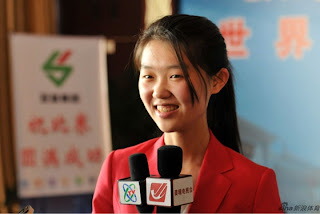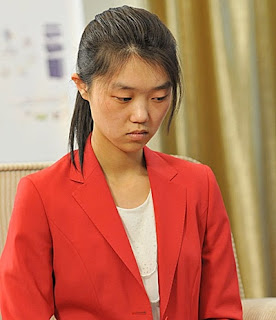## Saturday, May 19, 2012

### Blog On Hiatus

I'm announcing that UnlimitedGo is now on hiatus. I'm busy right now working on my undergraduate thesis and couldn't spend as much times as I could to browse and look at professional games. I will return perhaps at the last quarter of the year.

I'll see you again guys :)

## Sunday, April 8, 2012

### Xie Yimin Saved Japan

Wang Chenxing who single handedly eliminated Korea at the 2nd Huanglongshi Cup met the last member of team Japan, Xie Yimin. Xie is the last obstacle between China and the trophy of Huanglongshi Cup.Wang Chenxing (left) vs. Xe Yimin (right)

In this game, Xie played black while Wang played white. Xie went for a frontal attack starting from black 55. After black 61, the game went into a big fighting game. Xie showed her mastery in attacking and got a promising position. Black 117 was the deciding move and after Xie controlled the center completely, Wang resigned.Xie Yimin, the last defense of Japan.
With this, Japan still has their chance to win, though it is very hard and almost impossible. Let see if Xie can survive the next game and increase the chance or not.Wang Chenxing's winning streak finally ended. It seems that her red jacket didn't bring her good luck in this game.

Here is the game record:
Xie Yimin (black) vs. Wang Chenxing (white). Result: B+R

(;CA[Windows-1252]SZAP[MultiGo:4.4.4]EV[2nd Huanglongshi Cup, game 10]DT[2012-04-08]
PB[Xie Yimin ]BR[6p]PW[Wang Chenxing ]WR[5p]KM[7.5]HARE[B+R]MULTIGOGM
;B[qd];W[pp];B[dc];W[cp];B[ep];W[de];B[ce];W[cf];B[cd];W[eo];B[fo];W[do];B[fp];W[dq]
;B[fm];W[df];B[ed];W[dl];B[nq];W[qn];B[kp];W[od];B[mc];W[qc];B[qg];W[pd];B[rd];W[pf]
;B[qh];W[ld];B[lc];W[kd];B[kc];W[jd];B[ne];W[nd];B[md];W[me];B[jc];W[id];B[nf];W[nc]
;B[hb];W[rc];B[lg];W[jg];B[ji];W[ff];B[fk];W[fr];B[dk];W[ck];B[dj];W[el];B[cj];W[fl]
;B[gl];W[gm];B[gk];W[hm];B[gg];W[fi];B[fj];W[gi];B[gf];W[eh];B[ih];W[ij];B[ik];W[hh]
;B[ig];W[jj];B[hj];W[hi];B[ii];W[hg];B[if];W[hf];B[ge];W[he];B[le];W[dd];B[cc];W[fd]
;B[gd];W[ec];B[eb];W[fc];B[fe];W[ee];B[gc];W[fb];B[ea];W[ch];B[bg];W[bi];B[bj];W[bf]
;B[gb];W[bc];B[ed];W[ac];B[dh];W[dg];B[di];W[bh];B[eg];W[hp];B[ho];W[io];B[ip];W[go]
;B[hq];W[hn];B[gp];W[ho];B[gr];W[jp];B[jq];W[iq];B[ir];W[mp];B[ip];W[mq];B[fn];W[nr]
;B[kn];W[qj];B[mm];W[ec];B[oh];W[mk];B[kk];W[db];B[oj];W[ok];B[pk];W[pj];B[nk];W[ol]
;B[nj];W[og];B[ng])

### South Korea Eliminated From Huanglongshi Cup

"The 2nd Huanglongshi cup brought several surprises to the table. First, Wang Chenxing scored 7 consecutive victories. Second, Wang rewrite her winning streak from 7 to 8 as she beat Park Jieun at the 9th game. Third, as Park Jieun went down, South Korea lost all team member and eventually eliminated from the championship. Leaving only Japan and China competing for the title.Park Jieun (left) vs. Wang Chenxing (right)

Wang Chenxing who played black made a big framework at the bottom board at the beginning of the game. Park didn't rush to attack the framework and took care the center instead. Wang made a ko fight wth black 87. Park won the ko, but Wang got to play black 107 and 109 as a compensation. Later, Park won the second ko fight, but gave black a chance to solidify the center.Park Jieun, the last gate of team Korea

The game entered its endgame and black's position was solid enough to get through. I wonder if black 165 is better to be played at white 166 instead.Wang Chenxing promoted to 5 dan. I think this is the same case as Song Ronghui's promotion after 7th Jeongganjang cup

Here is the game record:
Wang Chenxing (black) vs. Park Jieun (white). Result: B+2.5

(;CA[Windows-1252]SZAP[MultiGo:4.4.4]EV[2nd Huanglongshi Cup, game 9]DT[2012-04-08]
PB[Wang Chenxing]BR[5p]PW[Park Jieun]WR[9p]KM[7.5]HARE[B+2.5]MULTIGOGM
;B[pd];W[dd];B[qp];W[dp];B[fq];W[cn];B[lq];W[qj];B[qf];W[qm];B[pn];W[ro];B[pm];W[rp]
;B[qq];W[ql];B[fo];W[dr];B[kn];W[in];B[ip];W[fc];B[dm];W[dn];B[em];W[bl];B[ej];W[kl]
;B[kj];W[ml];B[mn];W[ol];B[jc];W[pb];B[pc];W[ob];B[qb];W[rb];B[qc];W[lc];B[mb];W[lb]
;B[mc];W[md];B[nc];W[kd];B[ie];W[je];B[gc];W[id];B[he];W[ic];B[cd];W[ce];B[dc];W[cc]
;B[bd];W[db];B[de];W[ec];B[cf];W[ee];B[be];W[eg];B[nd];W[ch];B[jf];W[if];B[gf];W[ig]
;B[kf];W[me];B[gh];W[jh];B[mf];W[ne];B[ii];W[lh];B[nf];W[oe];B[ji];W[ki];B[lj];W[nh]
;B[lg];W[mi];B[kh];W[kg];B[jg];W[ih];B[kh];W[od];B[oc];W[kg];B[le];W[ke];B[kh];W[li]
;B[of];W[pf];B[pe];W[ld];B[pg];W[lf];B[ef];W[kg];B[fe];W[gd];B[cj];W[bj];B[bi];W[ck]
;B[aj];W[ak];B[bk];W[nn];B[nm];W[bj];B[hd];W[hc];B[bk];W[mm];B[ln];W[bj];B[fd];W[gb]
;B[bk];W[om];B[no];W[bj];B[qn];W[rn];B[bk];W[gg];B[fg];W[bj];B[jl];W[ai];B[jk];W[fr]
;B[eq];W[er];B[gr];W[fh];B[hg];W[fi];B[dh];W[gq];B[gp];W[ek];B[dk];W[dl];B[dj];W[el]
;B[cm];W[cl];B[bm];W[ci];B[cg];W[bh];B[fm];W[hq];B[hr];W[bn];B[an];W[fj];B[bp];W[dq]
;B[am];W[bq];B[gk];W[rq];B[pl];W[nl];B[pk];W[ri];B[qr];W[rg];B[aq];W[br];B[rf];W[hj]
;B[hk];W[rr];B[rs];W[ij];B[gj];W[hi];B[iq];W[eo];B[fp];W[jj];B[kk];W[km];B[jm];W[ik]
;B[il];W[eh];B[ed];W[dg];B[qh];W[pj];B[ok];W[nk];B[oj];W[oi];B[nj];W[mj];B[on];W[qo]
;B[po];W[rh];B[la];W[ka];B[ma];W[sf];B[se];W[sg];B[re];W[kb];B[bb];W[bc];B[ac];W[cb]
;B[bg];W[bo];B[ao];W[cp];B[ap];W[ba];B[ab];W[mg];B[nm];W[pp];B[oq];W[nn];B[lm];W[ll]
;B[nm];W[mk];B[qk];W[rk];B[ar];W[ag];B[af];W[fl];B[gl];W[en];B[fn];W[fs];B[gs];W[nn]
;B[bs];W[cs];B[gi];W[ni];B[nm];W[ir];B[hp];W[nn];B[oo];W[fk];B[ge];W[dc];B[ph];W[sr]
;B[df];W[ep];B[qg];W[oh];B[lk];W[aa];B[ss];W[ah];B[nn])

## Saturday, April 7, 2012

### Wang Chenxing Continues Her Rampage

The 2nd Huanglongshi Cup continues! After 2 months break, the female players from China, Japan, and Korea continues their competition to claim the best female team of the 3 professional countries. Wang Chenxing who scored 6 consecutive victories in the previous round continues her rampage by winning the 7th game from Mannami Nao of Japan.Wang Chenxing won her 7th game

The game was very calm. Both players focused on territory. I think white 106 already gave the victory to white. Black didn't get any chance to reverse the game afterward. A convincing victory by Wang Chenxing.Mannami Nao, the 4th member of team Japan

Here is the game record:
Wang Chenxing (white) vs. Mannami Nao (black). Result: W+R

(;
KM[7.5]
EV[2nd Huanglongshi Cup 8th round]
FF
SZ
GM
PW[Wang Chenxing]
WR[2d]
PB[Mannami Nao]
BR[2d]
RE[W+R]
DT[2012-04-06]
;B[pd];W[dd];B[qp];W[dq];B[oq];W[qf];B[nc];W[rd];B[qg];W[pg];B[qh]
;W[ph];B[rf];W[qe];B[qj];W[pi];B[qk];W[qc];B[kc];W[ck];B[gp];W[do]
;B[jp];W[rp];B[qq];W[qm];B[ok];W[qo];B[po];W[pn];B[ro];W[rn];B[qn]
;W[qi];B[ri];W[qo];B[cp];W[cq];B[qn];W[rj];B[qo];W[rk];B[rl];W[rh]
;B[sk];W[si];B[ql];W[fc];B[be];W[bd];B[ce];W[cd];B[ch];W[kn];B[jn]
;W[ko];B[kp];W[kl];B[jo];W[mo];B[km];W[lm];B[jm];W[ll];B[lp];W[bi]
;B[ik];W[ki];B[ci];W[bh];B[bg];W[cj];B[ei];W[ek];B[ah];W[bj];B[hc]
;W[om];B[rm];W[fq];B[gq];W[eg];B[ef];W[ff];B[ee];W[dg];B[cg];W[fe]
;B[gi];W[de];B[em];W[dm];B[en];W[dn];B[el];W[ej];B[fh];W[fr];B[dl]
;W[cl];B[pc];W[id];B[ic];W[kd];B[lc];W[hr];B[gr];W[gs];B[gd];W[ed]
;B[jh];W[kh];B[kg];W[lg];B[lf];W[mg];B[ji];W[jj];B[ij];W[mf];B[kj]
;W[lj];B[jk];W[nl];B[kf];W[md];B[qb];W[rb];B[qd];W[rc];B[ld];W[hf]
;B[gb];W[fb];B[ir];W[hq];B[is];W[hs];B[iq];W[hp];B[go];W[ho];B[gn]
;W[mp];B[mq];W[np];B[nq];W[gc];B[ep];W[dp];B[ie];W[hb];B[ib];W[ga]

Wang will play the last member of team Korea, Park Jieun (9 dan). Let see if Park can shot Wang down.Wang Chenxing (left) vs. Mannami Nao (right)

### Iyama Yuta Leads Judan by 2-1

The 50th Judan serves us a clash between 2 top Japanese players, Iyama Yuta, the title holder, and his challenger, Cho U. Iyama won the first game and took an early lead.

Iyama then won the second game on March 19 and got his game point. Iyama who played black kept the balance at the center well and after he converted the left side to his area, Cho U resigned.

Here is the game record:
Iyama Yuta (black) vs. Cho U (white). Result: B+R

(;
KM[6.5]
EV[50th Judan title match 2]
FF
SZ
GM
PW[Cho U]
WR[Kisei]
DT[2012-03-19]
BR[Judan]
RE[B+R]
PB[Iyama Yuta]
;B[pd];W[dp];B[qp];W[dc];B[op];W[ci];B[fq];W[dn];B[cq];W[dq];B[dr]
;W[er];B[cr];W[eq];B[bo];W[jq];B[cm];W[dm];B[cl];W[dl];B[jd];W[qf]
;B[nc];W[pj];B[ql];W[mq];B[ph];W[pl];B[pm];W[qk];B[ol];W[pk];B[qm]
;W[qh];B[fr];W[ds];B[co];W[do];B[ck];W[io];B[de];W[ce];B[cf];W[cd]
;B[df];W[ej];B[dj];W[fc];B[di];W[or];B[gn];W[gp];B[fp];W[go];B[fo]
;W[fn];B[fm];W[en];B[hn];W[in];B[lq];W[lr];B[mr];W[nr];B[kr];W[ms]
;B[ip];W[iq];B[jp];W[kq];B[ho];W[hq];B[ek];W[fl];B[el];W[gm];B[hp]
;W[gq];B[em];W[hm];B[ko];W[hk];B[dk];W[ln];B[lo];W[mo];B[im];W[il]
;B[jm];W[kl];B[mn];W[nn];B[km];W[mm];B[ll];W[kk];B[lm];W[lj];B[ml]
;W[nj];B[mi];W[mj];B[ij];W[jl];B[cs];W[es];B[nm];W[of];B[qe];W[rf]
;B[dd];W[cc];B[gr];W[hr];B[fs];W[hs];B[ne];W[ic];B[jc];W[nf];B[mf]
;W[oe];B[me];W[od];B[oc];W[jb];B[kb];W[ib];B[re];W[lb];B[ka];W[on]
;B[om];W[nk];B[mn];W[pc];B[qc];W[mm];B[nl];W[pb];B[lc];W[qb];B[pe]
;W[rc];B[pf];W[oh];B[pg];W[pi];B[rd];W[sc];B[qg];W[rh];B[rg];W[og]
;B[sd];W[sf];B[rb];W[qd];B[qi];W[ri];B[qc];W[kp];B[jo];W[qd];B[rj]
;W[rk];B[qc];W[qo];B[sb];W[po];B[mn];W[rn];B[mp];W[mm];B[gl];W[hl]
;B[mn];W[nd];B[pp];W[mm];B[oo];W[pn];B[lp];W[rp];B[nq];W[jr];B[mr]
;W[ks];B[qq];W[rq];B[rr];W[pr];B[qr];W[rm];B[sr];W[mc];B[mb];W[md]
;B[nb];W[ld];B[kc];W[bf];B[bg];W[be];B[ch];W[kf];B[gj];W[gs];B[cp]
;W[eo];B[rl];W[sl];B[gk];W[jh];B[ke];W[le];B[ih];W[ig];B[hg];W[ii]
;B[hh];W[ji];B[gd];W[fd];B[fe];W[hi];B[gc];W[gb];B[fb];W[eb];B[gi]
;W[gf];B[if];W[hf];B[jg];W[ge];B[kg];W[lg];B[ed];W[ec];B[gg];W[ff]
;B[fg])

The third game was played on April 5. Cho U faced a must win situation as he was behind by 0-2. If he lost the third game, his chance to claim Judan would be 0. Fortunately, he won this game and the match went to its 4th game. Cho got a nice attacking game this time. He threw 11 stones away at the upper left corner to capture Iyama's group at the center. Cho solidified his center and Iyama resigned soon afterward.Iyama Yuta (left) vs. Cho U (right)

Here is the game record:
Cho U (black) vs. Iyama Yuta (white). Result: B+R

(;
KM[6.5]
EV[50th Judan title match 3]
FF
SZ
GM
PB[Cho U]
DT[2012-04-05]
BR[Kisei]
RE[B+R]
PW[Iyama Yuta]
WR[Judan]
;B[qd];W[dd];B[pq];W[dp];B[fc];W[cf];B[kc];W[po];B[qm];W[qq];B[mq]
;W[pp];B[pr];W[np];B[lq];W[qn];B[pl];W[qr];B[iq];W[qf];B[pe];W[rd]
;B[re];W[qe];B[rf];W[pd];B[qc];W[pf];B[oe];W[rc];B[rb];W[of];B[ne]
;W[nf];B[mf];W[me];B[oc];W[pc];B[qb];W[rg];B[sc];W[mg];B[lf];W[pj]
;B[lg];W[mh];B[lh];W[mi];B[qh];W[sf];B[sd];W[qg];B[qj];W[pi];B[rn]
;W[ro];B[pn];W[qo];B[qk];W[kd];B[jd];W[ke];B[id];W[md];B[nc];W[jf]
;B[li];W[ig];B[mj];W[nj];B[ii];W[lj];B[mk];W[kj];B[jh];W[nk];B[ml]
;W[nl];B[mm];W[nm];B[rl];W[sn];B[rh];W[jk];B[hj];W[rm];B[ph];W[oh]
;B[sh];W[sg];B[pk];W[on];B[pm];W[ge];B[ln];W[gc];B[gd];W[hd];B[fd]
;W[hc];B[he];W[fb];B[eb];W[gb];B[hf];W[fe];B[ee];W[ff];B[dc];W[cc]
;B[cd];W[de];B[cb];W[bc];B[ec];W[bb];B[ef];W[fg];B[ed];W[ie];B[cg]
;W[ce];B[eg];W[fh];B[hg];W[hh];B[ih];W[gi];B[hi];W[dh];B[ib];W[gg]
;B[gh];W[nd];B[fi];W[eh];B[gj];W[dg];B[od];W[ea];B[fp];W[dn];B[fn]
;W[fq];B[gq];W[eq];B[gr];W[mn];B[mo];W[mp];B[lp];W[no];B[nn];W[ki]
;B[kh];W[mn];B[km])

The 4th game will be played on April 18. Iyama will play for his title, while Cho U will face another must win game. Who will come out as the winner?The happy Cho U survived the 3rd game.

## Sunday, April 1, 2012

### Iyama Yuta the 67th Honinbo's Challenger

Honinbo is 1 of the top 3 Japanese title. It has a long history and could be dated back in Edo period. After it became an annual tournament, 16 people already claimed this title. This year, Iyama Yuta got his first shot to be the 17th people to hold the annual Honinbo.

Iyama won the Honinbo league in his 3rd try. In the previous 2 leagues, he scored 4-3 and 6-1 and stayed at the second position. This year he scored 6-1 again but the circumstance is difference as he collected most win and doesn't have to play a tiebreak game.

Iyama won the last game against Hane Naoki and avoided a possible tie result with Yuki Satoshi. This victory gave Iyama his 5th major title challenger's right. Oza and Gosei are the only tournament where he never become challenger.Hane Naoki (left) vs. Iyama Yuta (right)
Final league result:
1. Iyama Yuta 6-1
2. Yuki Satoshi 5-2
3. Cho U 4-3
4. Takao Shinji & Kono Rin 4-3 (Will play demotion player decision match)
6. Hane Naoki 3-4 (demoted)
7-8 Seto Taiki & Ko Iso 1-6 (demoted)

Here is one of Iyama game during the league. His second round's victory from Cho U started his winning streak at the league after previously losing to Takao Shinji at the first round.

Here is the game record:
Iyama Yuta (white) vs. Cho U (black). Result: W+R

(;
WR[Judan]
EV[67th Honinbo league]
FF
SZ
PW[Iyama Yuta]
GM
PB[Cho U]
BR[Kisei]
RE[W+R]
DT[2011-11-14]
KM[6.5]
;B[pd];W[dp];B[qp];W[dc];B[lq];W[nc];B[pf];W[jd];B[df];W[ce];B[cn]
;W[dk];B[fp];W[dn];B[dm];W[en];B[em];W[co];B[cm];W[fn];B[fm];W[gn]
;B[di];W[hq];B[cq];W[bp];B[er];W[gm];B[bq];W[dj];B[ci];W[bj];B[fk]
;W[fl];B[el];W[gl];B[ek];W[ej];B[fh];W[fi];B[gi];W[eh];B[gj];W[fg]
;B[gh];W[ei];B[eg];W[dg];B[ef];W[cf];B[gf];W[fq];B[eq];W[fr];B[gp]
;W[ep];B[hp];W[es];B[dr];W[iq];B[ip];W[jp];B[jo];W[io];B[jq];W[kp]
;B[ir];W[hr];B[hs];W[gr];B[kr];W[ho];B[lp];W[ko];B[lo];W[qk];B[gs]
;W[fo];B[kn];W[jn];B[kq];W[jo];B[ds];W[go];B[ql];W[qq];B[rp];W[pp]
;B[qn];W[oq];B[oo];W[po];B[pn];W[rr];B[nr];W[or];B[rq];W[pr];B[sr]
;W[on];B[no];W[qs];B[op];W[nq];B[mr];W[os];B[qi];W[qc];B[pc];W[pb]
;B[lc];W[qd];B[qe];W[oe];B[pe];W[ob];B[le];W[gd];B[re];W[kc];B[kb]
;W[jb];B[kd];W[jc];B[rb];W[qb];B[sb];W[ke];B[ld];W[lb];B[mb];W[ka]
;B[mc];W[ma];B[na];W[nb];B[la];W[lf];B[kf];W[je];B[mf];W[lg];B[mg]
;W[me];B[lh];W[kg];B[kh];W[jg];B[ne];W[ma];B[md];W[hg];B[gg];W[oa]
;B[bk];W[bl];B[ck];W[cj];B[cl];W[ll];B[mk];W[nd];B[me];W[ml];B[nl]
;W[mn];B[nn];W[nm];B[om];W[nk];B[mm];W[mj];B[cc];W[dd];B[jh];W[mh]
;B[ig];W[jf];B[of];W[km];B[db];W[bb];B[cd];W[cb];B[de];W[ee];B[ff]
;W[bd];B[fd];W[ok];B[lk];W[kj];B[kk];W[jk];B[kl];W[lm];B[jl];W[jj]
;B[il];W[hk];B[lj];W[li];B[oi];W[mi];B[rs];W[qj];B[ri];W[ss];B[nj]
;W[pk];B[rs];W[gq];B[fs];W[ss];B[ii];W[ki];B[rs];W[js];B[qr];W[is]
;B[es];W[rr];B[rk];W[ss];B[oj];W[jm];B[rs];W[jr];B[bs];W[ss];B[ol]
;W[im];B[rs];W[fc];B[gc];W[ss];B[rj];W[ik];B[rs];W[fe];B[ge];W[ed]
;B[hd];W[fd];B[hb];W[ss];B[fb];W[bc];B[rs];W[if];B[he];W[ss];B[pl]
;W[hl];B[rs];W[ng];B[nf];W[ss];B[dh];W[cg];B[rs];W[oh];B[ph];W[ss]
;B[bi];W[ai];B[rs];W[gb];B[ga];W[ss];B[bh];W[bg];B[rs];W[ic];B[hc]
;W[ss];B[ah];W[aj];B[rs];W[cr])

## Tuesday, March 20, 2012

### Cho U Defended Kisei

The 36th Kisei-sen met its climax at the 7th game. Takao Shinji won the critical 6th game and got his final chance to capture the title. Cho played black in this game, while Takao got white.Takao Shinji (left) vs. Cho U (right)

Cho opened the game with a big framework at the right side. Takao made an invasion with white 46. Cho got an attack at the bottom board for Takao's success invasion. He sacrificed 2 stones at the top to win the ko. Later, attacking white's formation at the bottom gave black enough point on the left side. Takao resigned after 281 moves.

This is the third year for Cho U as a Kisei. Previously he captured the title from Yamashita Keigo and defended it well from Iyama Yuta.

Here is the game record:
Cho U (black) vs. Takao Shinji (white). Result: B+R

(;
KM[6.5]
EV[36th Kisei title match 1]
FF
SZ
GM
PW[Takao Shinji]
WR[9d]
PB[Cho U]
BR[Kisei]
RE[B+R]
DT[2012-03-14,15]
;B[qd];W[dp];B[pp];W[dc];B[lc];W[qn];B[pj];W[nq];B[oo];W[qq];B[qp]
;W[pq];B[rq];W[lp];B[ol];W[od];B[pf];W[qc];B[rc];W[md];B[ld];W[pc]
;B[rb];W[le];B[ke];W[lf];B[kf];W[mc];B[jc];W[nf];B[ph];W[ce];B[fq]
;W[hq];B[cq];W[dq];B[cp];W[do];B[dr];W[er];B[cr];W[eq];B[cn];W[gc]
;B[rr];W[qk];B[qj];W[pm];B[mq];W[mp];B[om];W[rj];B[ri];W[rl];B[oq]
;W[or];B[op];W[si];B[rh];W[ro];B[nr];W[mr];B[np];W[lq];B[pl];W[ql]
;B[pn];W[qm];B[lr];W[nq];B[sh];W[sj];B[mq];W[kd];B[jd];W[nq];B[sm]
;W[sn];B[mq];W[lb];B[ms];W[kc];B[jq];W[ho];B[ge];W[ee];B[hc];W[hb]
;B[gb];W[hd];B[ic];W[gd];B[fb];W[eb];B[ie];W[jr];B[dn];W[jp];B[fn]
;W[ci];B[fc];W[fd];B[ec];W[db];B[gp];W[fp];B[fo];W[hr];B[fr];W[fs]
;B[ep];W[gr];B[ck];W[kg];B[jg];W[hg];B[kh];W[lg];B[if];W[ei];B[jo]
;W[kq];B[ko];W[hp];B[ln];W[jh];B[ih];W[ji];B[ig];W[lj];B[fg];W[eg]
;B[hn];W[eo];B[bi];W[bj];B[io];W[fp];B[sp];W[sl];B[ep];W[kr];B[ip]
;W[fp];B[so];W[rn];B[ep];W[iq];B[kp];W[fp];B[cj];W[bh];B[ep];W[in]
;B[en];W[fp];B[di];W[ch];B[ep];W[hm];B[gn];W[fp];B[ki];W[kj];B[ep]
;W[lo];B[km];W[fp];B[rk];W[sk];B[ep];W[mn];B[mm];W[fp];B[ii];W[jj]
;B[ep];W[ll];B[nn];W[fp];B[mi];W[li];B[ep];W[mo];B[im];W[fp];B[mh]
;W[lh];B[ep];W[lm];B[bk];W[ed];B[ib];W[ai];B[fp];W[ml];B[jn];W[nm]
;B[ej];W[dh];B[dj];W[pd];B[qe];W[hk];B[fi];W[qb];B[hj];W[ik];B[gk]
;W[gl];B[fl];W[ff];B[gg];W[rp];B[sq];W[ak];B[co];W[es];B[al];W[aj]
;B[bl];W[ra];B[gf];W[ps];B[ns];W[no];B[on];W[ij];B[gj];W[og];B[nk]
;W[pk];B[ok];W[he];B[hf];W[pg];B[qg];W[mj];B[nj];W[oh];B[oi];W[eh]
;B[mg];W[mf];B[pe];W[fh];B[gh];W[kl];B[ka];W[kb];B[ja];W[la];B[kn]
;W[ks];B[ls];W[gs];B[il];W[hl];B[jl];W[ds];B[cs];W[gm];B[nl];W[fm]
;B[em];W[jb];B[ha];W[fa];B[mm];W[qr];B[rs];W[nm];B[fe];W[ef];B[mm]
;W[sr];B[os];W[nm];B[oe];W[ne];B[mm])